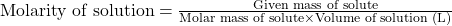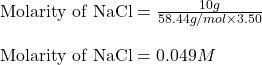## What is the molarity of a solution that is composed of 10 grams of Sodium Chloride ( NaCl) dissolved in 3.50 L of water

Question

What is the molarity of a solution that is composed of 10 grams of Sodium Chloride ( NaCl) dissolved in 3.50 L of water

in progress 0
6 months 2021-07-29T09:26:15+00:00 1 Answers 5 views 0

1. Answer: The molarity of solution will be 0.049 M

Explanation:

Molarity is defined as the amount of solute expressed in the number of moles present per liter of solution. The units of molarity are mol/L. The formula used to calculate molarity:We are given:

Given mass of sodium chloride = 10 g

Molar mass of sodium chloride = 58.44 g/mol

Volume of solution = 3.50 L

Putting values in above equation:Hence, the molarity of solution will be 0.049 M